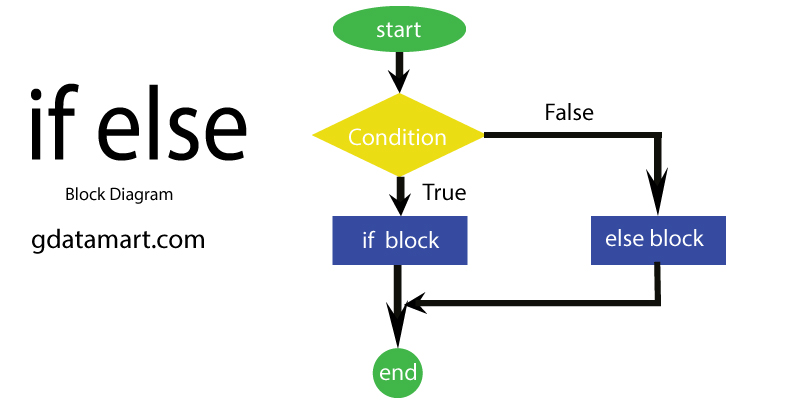# What is if else in C Programming

Author: Janet

## if else in C

In C programming if-else statement is an extended form of an if statement, In an if-else statement there is one condition to check, and on the basis of its result, one decision is selected out of two decisions.

In if-else there are:

• ONE condition
• TWO possible decisionsIf the condition is true then if-block will be executed and if the condition is false then else-block will be executed.

#### if-else Statement Syntax

if(condition)

{

// if-block codes

}

else

{

// else-block codes

}

The working of the if-else statement is very simple, In the above syntax if the condition is true then if-block codes will be executed and if the condition is false then else-block codes will be executed.

### if-else Statement  Examples

#### Example 1:

Program: To find the greater number in two numbers

#include<stdio.h>

int main()

{

int A=27, B=34;

if(A>B)

{

printf("A is greater than B \n");

}

else

{

printf("B is greater than A \n");

}

return 0;

}

#### Output:

B is greater than A

**************************************************

#### Example 2:

Program: To find out the given number is an even number or an odd number

#include<stdio.h>

int main()

{

int num=34;

if(num % 2==0)

{

printf("%d is an Even Number",num);

}

else

{

printf("%d is an Odd Number",num);

}

return 0;

}

#### Output:

34 is an Even Number